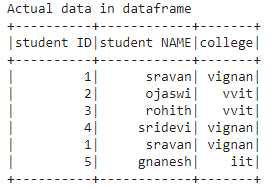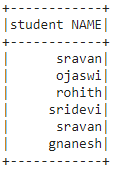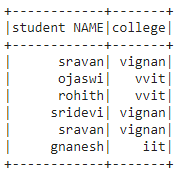# Select specific column of PySpark dataframe with its position

• Last Updated : 08 Oct, 2021

In this article, we will discuss how to select a specific column by using its position from a pyspark dataframe in Python. For this, we will use dataframe.columns() method inside dataframe.select() method.

Syntax:

dataframe.select(dataframe.columns[column_number]).show()

where,

• dataframe is the dataframe name
• dataframe.columns[]: is the method which can take column number as an input and select those column
• show() function is used to display the selected column

Let’s create a sample dataframe.

## Python3

 `# importing module``import` `pyspark` `# importing sparksession from pyspark.sql module``from` `pyspark.sql ``import` `SparkSession` `# creating sparksession and giving an app name``spark ``=` `SparkSession.builder.appName(``'sparkdf'``).getOrCreate()` `# list  of students  data``data ``=` `[[``"1"``, ``"sravan"``, ``"vignan"``], [``"2"``, ``"ojaswi"``, ``"vvit"``],``        ``[``"3"``, ``"rohith"``, ``"vvit"``], [``"4"``, ``"sridevi"``, ``"vignan"``],``        ``[``"1"``, ``"sravan"``, ``"vignan"``], [``"5"``, ``"gnanesh"``, ``"iit"``]]` `# specify column names``columns ``=` `[``'student ID'``, ``'student NAME'``, ``'college'``]` `# creating a dataframe from the lists of data``dataframe ``=` `spark.createDataFrame(data, columns)` `print``(``"Actual data in dataframe"``)` `# show dataframe``dataframe.show()`

Output:Selecting a column by column number

## Python3

 `# select column with column number 1``dataframe.select(dataframe.columns[``1``]).show()`

Output:We can also select multiple columns with the same function with slice operator(:). It can access up to n columns.

Syntax: dataframe.select(dataframe.columns[column_start:column_end]).show()

## Python3

 `#select column with column number slice operator``dataframe.select(dataframe.columns[``1``:``3``]).show()`

Output:My Personal Notes arrow_drop_up